##全国校区# 可视化工具不知道怎么选？深度评测5大python数据可视化工具2020-07-29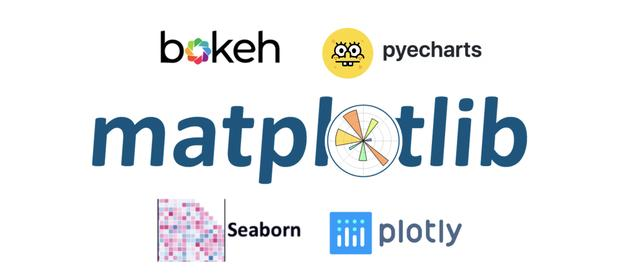# 指标说明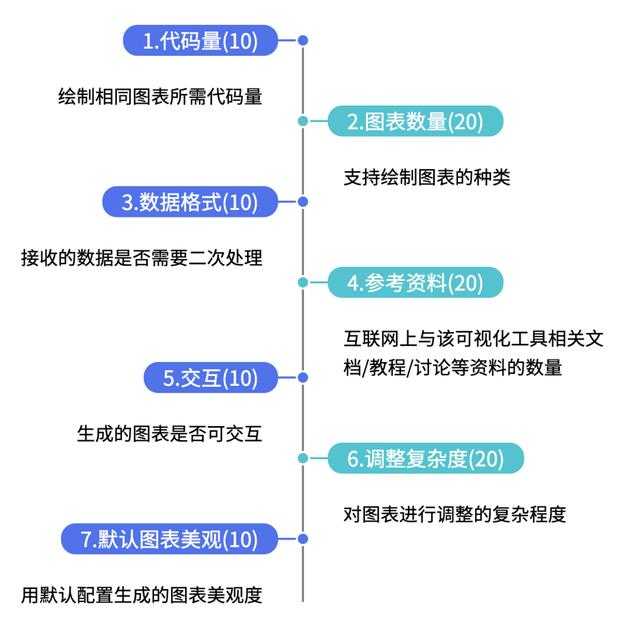# 数据说明

```from pyecharts.faker import Faker
x = Faker.choose()
y1 = Faker.values()
y2 = Faker.values()```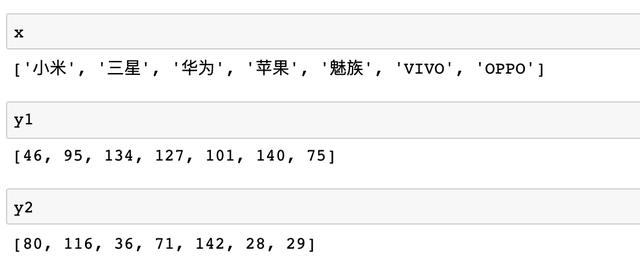x为一列品牌名称，y1/y2为一列相同长度的无意义数据，接下来让我们使用不同的库对这组数据进行可视化！

01Pyecharts

Echarts是一个由百度开源的数据可视化，凭借着良好的交互性，精巧的图表设计，得到了众多开发者的认可。而Python是一门富有表达力的语言，很适合用于数据处理。当数据分析遇上数据可视化时，pyecharts 诞生了，支持30+种图表。在pyecharts中制作条形图首先需要导入相关库

```from pyecharts import options as opts
from pyecharts.charts import Bar```

```c = (
Bar()
.set_global_opts(title_opts=opts.TitleOpts(title="Pyecharts—柱状图", subtitle=""))
).render_notebook()```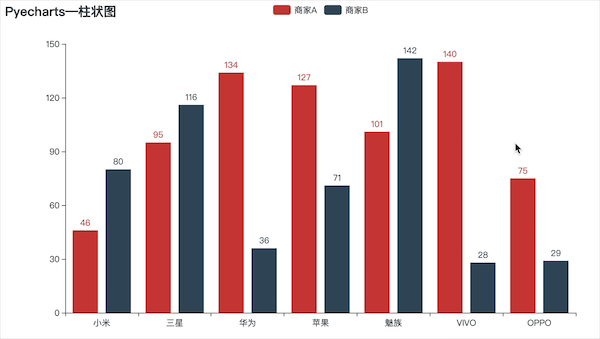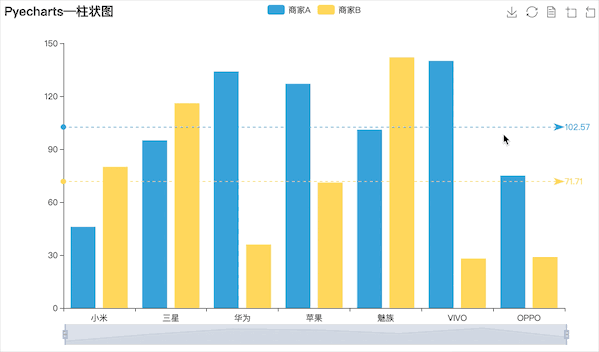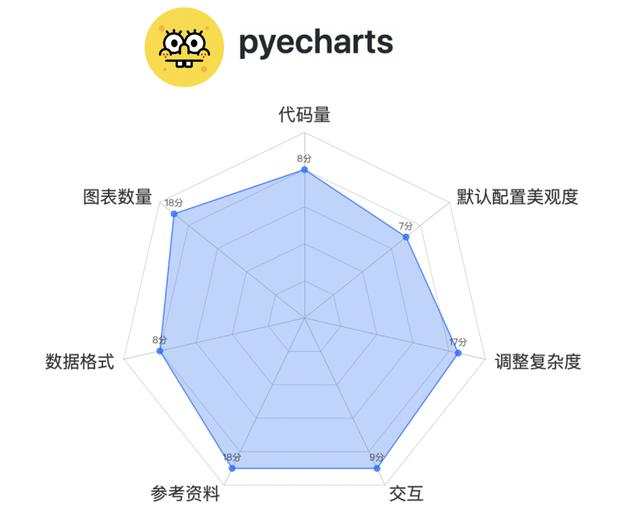02Matplotlib

Matplotlib应该是最广泛使用的Python可视化工具，支持的图形种类非常多，使用Matplotlib制作相同效果的图需要先导入相关库，并且并不支持原生中文所以还要设置下中文显示

```import matplotlib.pyplot as plt
import numpy as np
plt.rcParams['font.sans-serif'] = ['SimHei'] ```

```width = 0.35
x1 = np.arange(len(x))

fig, ax = plt.subplots()
rects1 = ax.bar(x1 - width/2, y1, width, label='商家A')
rects2 = ax.bar(x1 + width/2, y2, width, label='商家B')

ax.set_title('Matplotlib—柱状图')
ax.set_xticks(x1)
ax.set_xticklabels(x)
ax.legend()

plt.show()```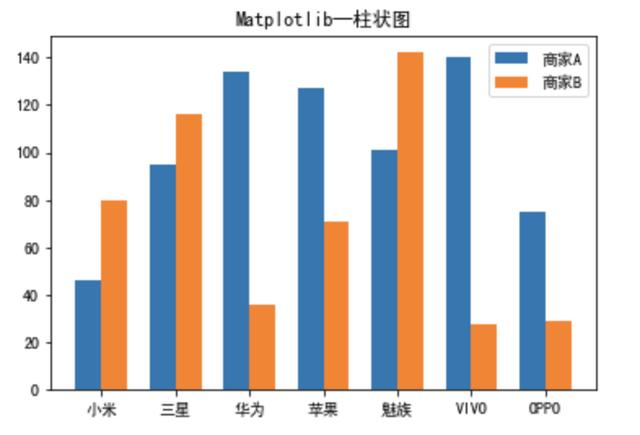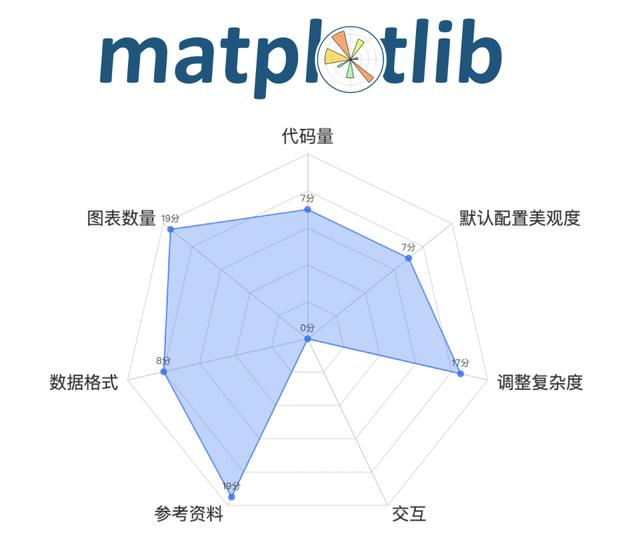03Plotly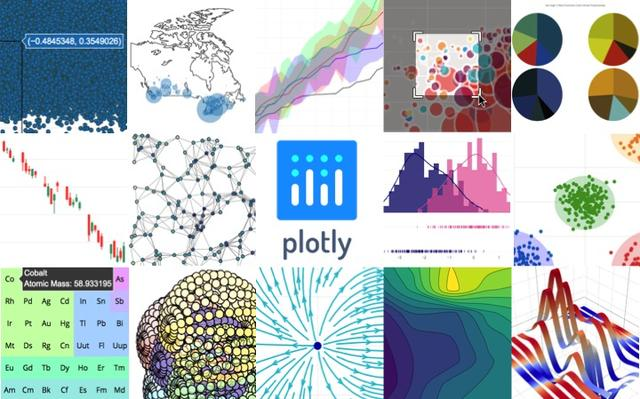Plotly也是一款非常强大的Python可视化库，Plotly内置完整的交互能力及编辑工具，支持在线和离线模式，提供稳定的API以便与现有应用集成，既可以在web浏览器中展示数据图表，也可以存入本地拷贝。但是由于官方未提供中文文档，网上关于Plotly的教程也仅限于官方的一些demo，对于一些详细的参数设置并没有太多资料，首先还是先导入相关库并设置notebook显示

```import plotly
import plotly.offline as py
import plotly.graph_objs as go
plotly.offline.init_notebook_mode(connected=True)```

```trace0 = go.Bar(
x = x,
y = y1,
name = '商家A',
)
trace1 = go.Bar(
x = x,
y = y2,
name = '商家B',
)
data = [trace0,trace1]
layout = go.Layout(
title={
'text': "Plotly-柱状图",
'y':0.9,
'x':0.5,
'xanchor': 'center',
'yanchor': 'top'})
fig = go.Figure(data=data, layout=layout)
py.iplot(fig)```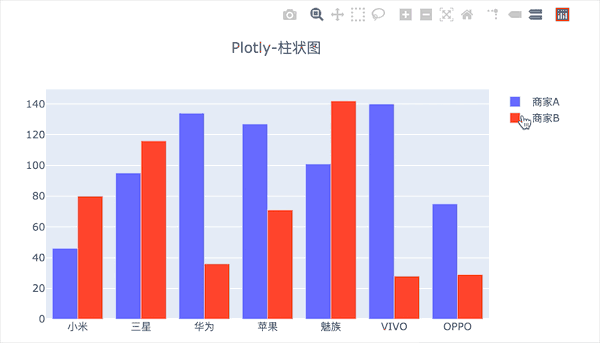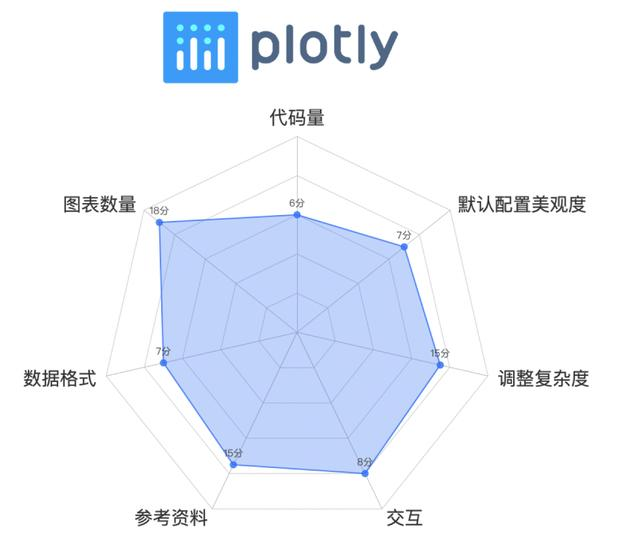04Bokeh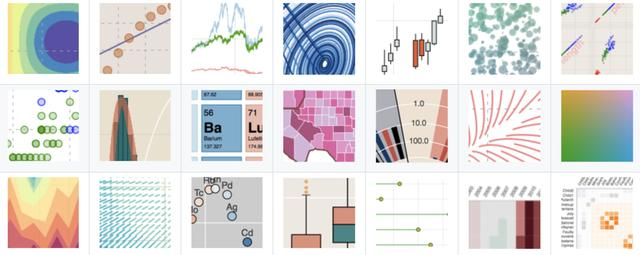Bokeh是一个专门针对Web浏览器的呈现功能的交互式可视化Python库。这是Bokeh与其它可视化库最核心的区别，它可以做出像D3.js简洁漂亮的交互可视化效果，但是使用难度低于D3.js，首先还是导入相关库

```from bokeh.transform import dodge
import pandas as pd
from bokeh.core.properties import value
import numpy as np
import matplotlib.pyplot as plt
%matplotlib inline
from bokeh.io import output_notebook
output_notebook() # 导入notebook绘图模块
from bokeh.plotting import figure,show
from bokeh.models import ColumnDataSource# 导入图表绘制、图标展示模块 # 导入ColumnDataSource模块 # 导入dodge、value模块```

```df = pd.DataFrame({'商家A':y1,'商家B':y2},
index = x_)
_x = ['商家A','商家B']    # 系列名
data = {'index':x_}
for i in _x:
data[i] = df[i].tolist()# 生成数据，数据格式为dict
source = ColumnDataSource(data=data)# 将数据转化为ColumnDataSource对象

p = figure(x_range=x_, y_range=(0, 150), plot_height=350, title="boken-柱状图",tools="crosshair,pan,wheel_zoom,box_zoom,reset,box_select,lasso_select")

p.vbar(x=dodge('index', -0.1, range=p.x_range), top='商家A', width=0.2, source=source,color="#718dbf", legend=value("商家A"))
p.vbar(x=dodge('index',  0.1, range=p.x_range), top='商家B', width=0.2, source=source,color="#e84d60", legend=value("商家B"))# dodge(field_name, value, range=None) → 转换成一个可分组的对象，value为元素的位置（配合width设置）
p.xgrid.grid_line_color = None
p.legend.location = "top_left"
p.legend.orientation = "horizontal" # 其他参数设置
show(p)```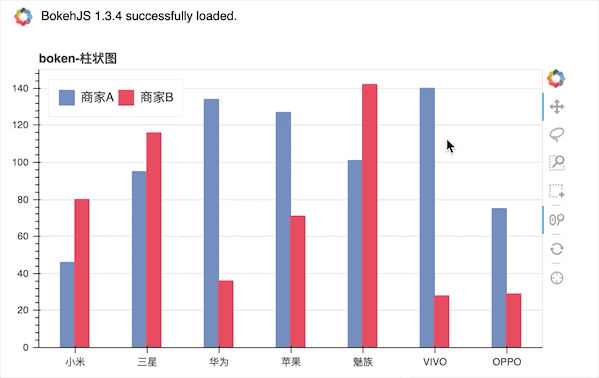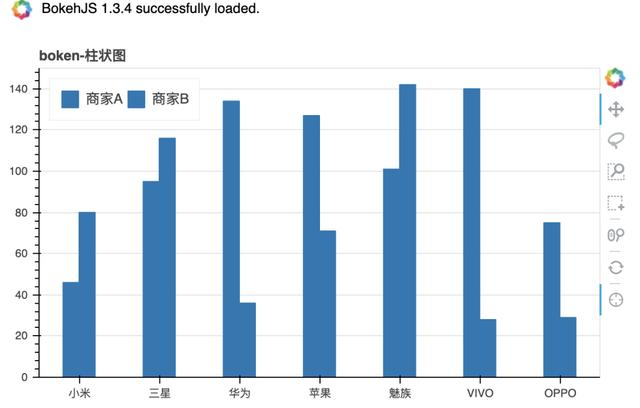Bokeh一个很明显的特点就是代码量较上面三个工具要多了很多，大多是在数据的处理上，并且和Plotly一样，有关bokeh相关的中文资料也不多，大多是入门型的基本使用于介绍，虽然从官方给出的图来看能作出很多比pyecharts更精美的图，但是查找相关参数的设置上将会耗费一定时间，主观评分71分。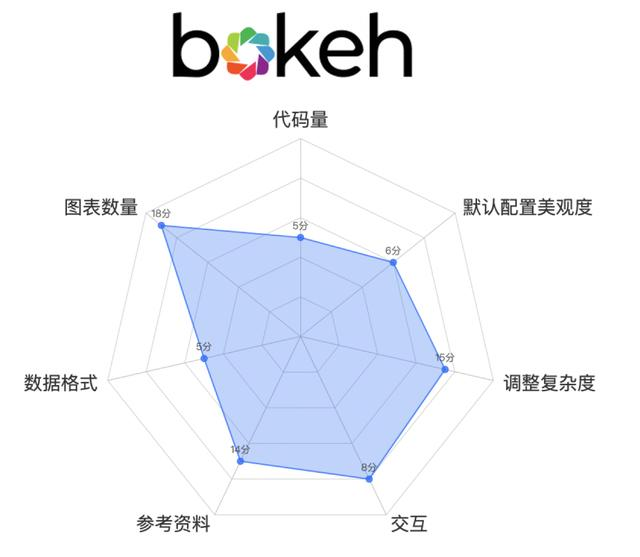05Seaborn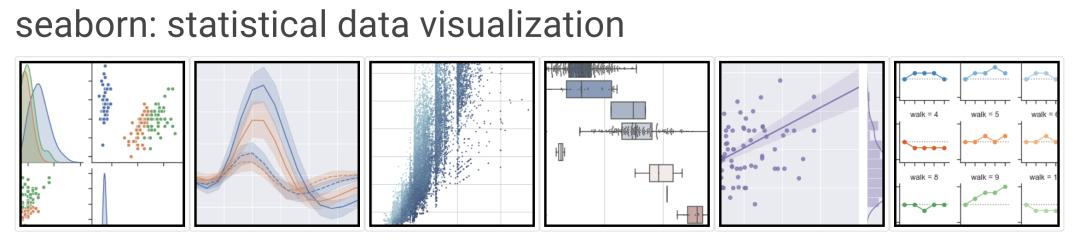seaborn官网给出的标题就知道，seaborn是为了统计图表设计的，它是一种基于matplotlib的图形可视化库，也就是在matplotlib的基础上进行了更高级的API封装，从而使得作图更加容易，在大多数情况下使用seaborn就能做出很具有吸引力的图，而使用matplotlib就能制作具有更多特色的图，还是我们的数据，使用Seaborn制作首先需要导入相关库，由于是基于Matplotlib，所以还是需要设置中文

```import seaborn as sns
import matplotlib.pyplot as plt
plt.rcParams['font.sans-serif'] = ['SimHei'] ```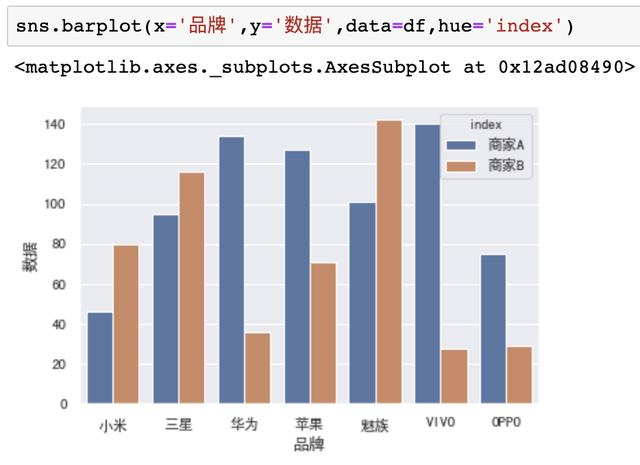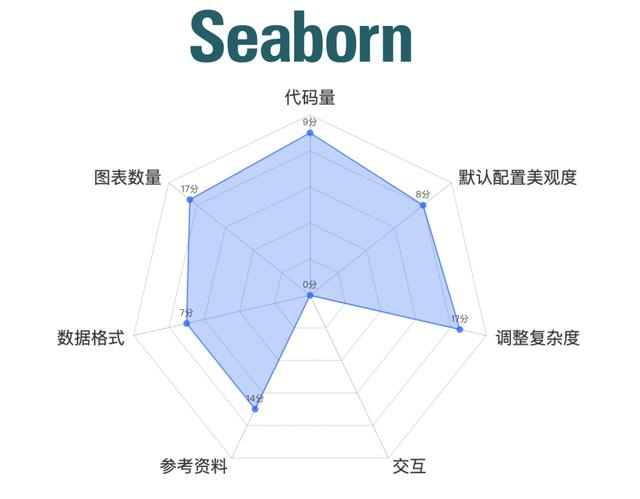# 小结

OK